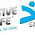## 2013年5月16日 星期四

### Arduino範例13：Get frequency from Optical encoder by Arduino (量測encoder的訊號)

In past articles, we know how to control a small DC motor by PWM signal.

### 直流馬達的速控 (二) : 使用Ardunio產生PWM 驅動馬達

But if we want to do feedback control, we should know rotation speed.
So today, I practice using Arduino to get frequency from motor.

Below picture is a small DC motor with optical encoder.
This is tear down from inkjet printer.

You can see that, it has 448 "line" per Revolution, so I could get 448 pulses in every one turn.

Below is the wire of connecting line. 2 of the left are power for DC motor, 4 of the right are for optical encoder. We should give 5V & GND to encoder, but also get 2 signals from it. the 2 signal could let us known the direction of rotation.
Ok, let me connecting the wire to Arduino board, get 1 signal on pin 2, and output PWM from pin 12. And also use 2003 to amplify the current of PWM to motor.

Code
I have no background of software program, so the code isn't concise. However, I am super happy for first time I wrote down it without example.

//the thought of code is "get every 100 pulses time, and average them.
int val = 0; // variable of pin reading status

int counter = 0;
int m = 0;
int i = 0;
float sumcycle;
float precycle;

void setup() {
pinMode(2, INPUT); // declare pin 2 as input
Serial.begin(9600);
}

void loop(){
if (val == HIGH) {
m = 1;
}
else {
m = 0;
}
if(m != i){
if(m == 1){
counter = counter + 1;
float halfcycle = pulseIn(2, LOW);
sumcycle = 2*halfcycle + precycle;
precycle = sumcycle;

if (counter ==100){
float fq = 100000000/448/precycle;
Serial.println(fq);
counter =0;
precycle =0;
}
}
}
i = m;
}

Video

Summery
There are so many ways could get frequency by Arduino.
The next step is using this frequency signal to do PID control.

#### 8 則留言:

1.gracias muy util saludos de México!

1.Hola, y gracias por su respuesta

2.sketch_jun25a.ino: In function 'void loop()':
sketch_jun25a:13: error: 'val' was not declared in this scope

3.You didn't write "int val = 0; "

4.hi, may i know why RPM is calculate as 100000000/448/precycle?
i dont understand this part...?

thanks

1.2.because precycle is in microseconds
the writter change it into seconds so 100 become 100*10^6

5.Hi! Your pages helped me control a motor I salvaged from an inkjet printer! Thanks so much!